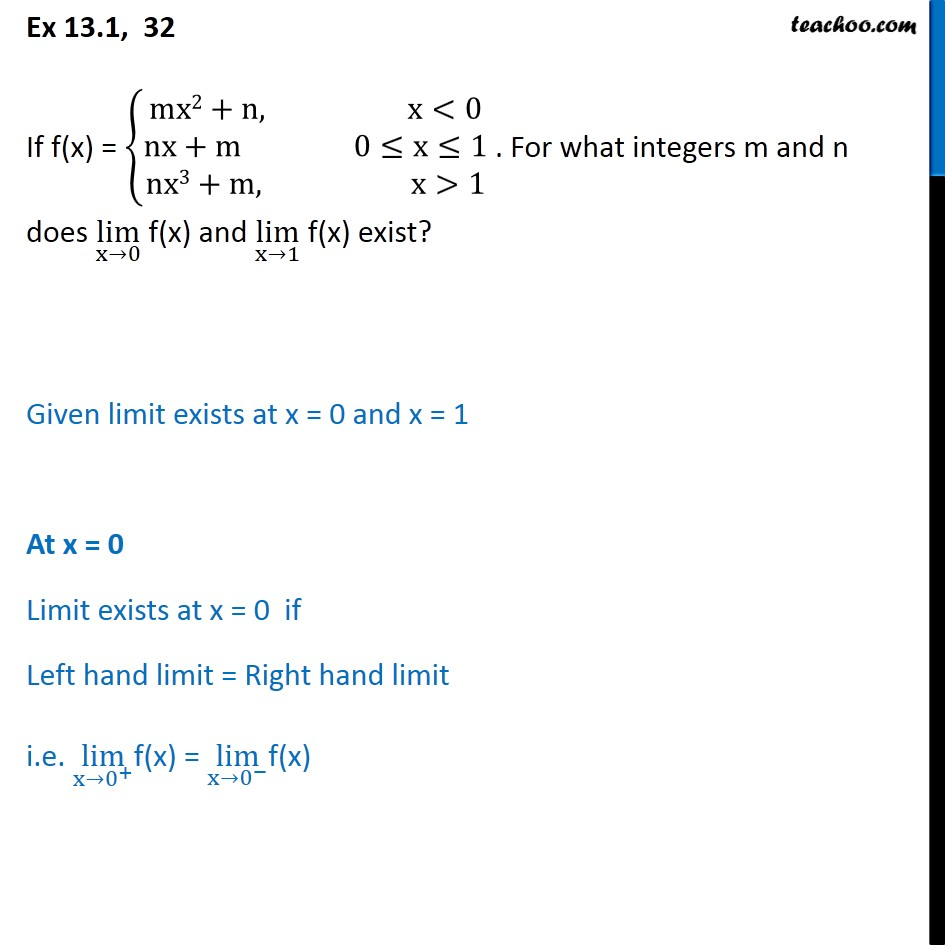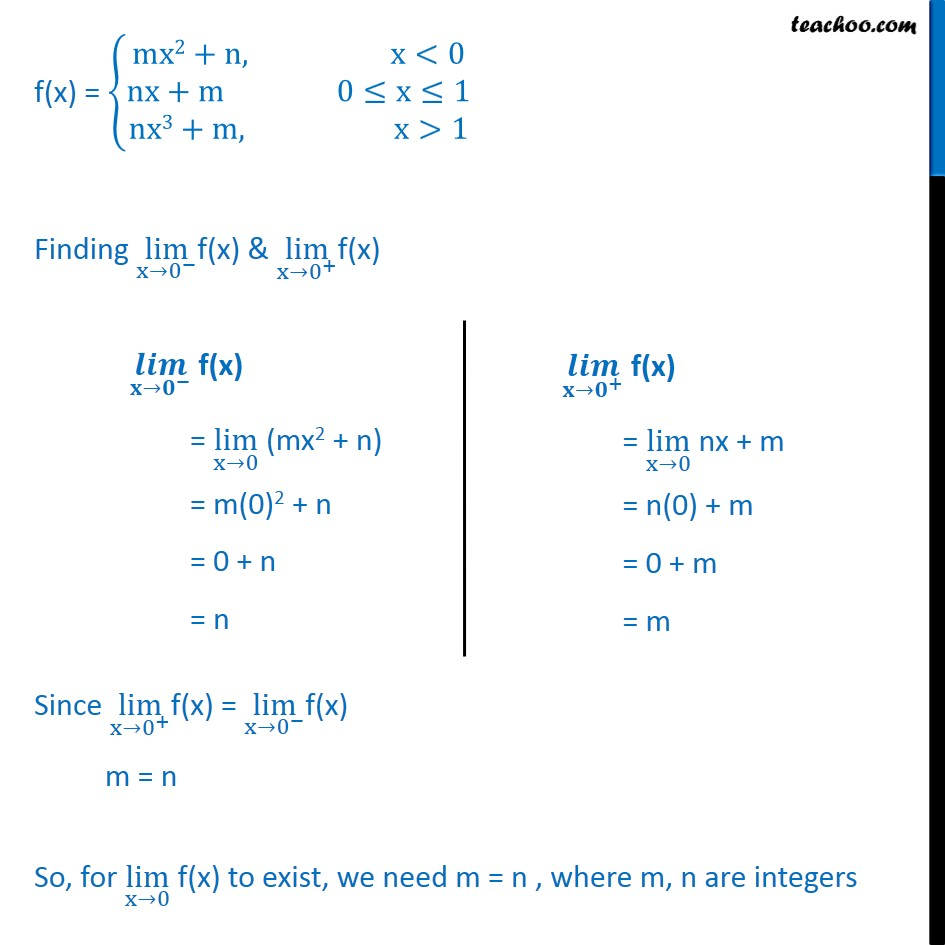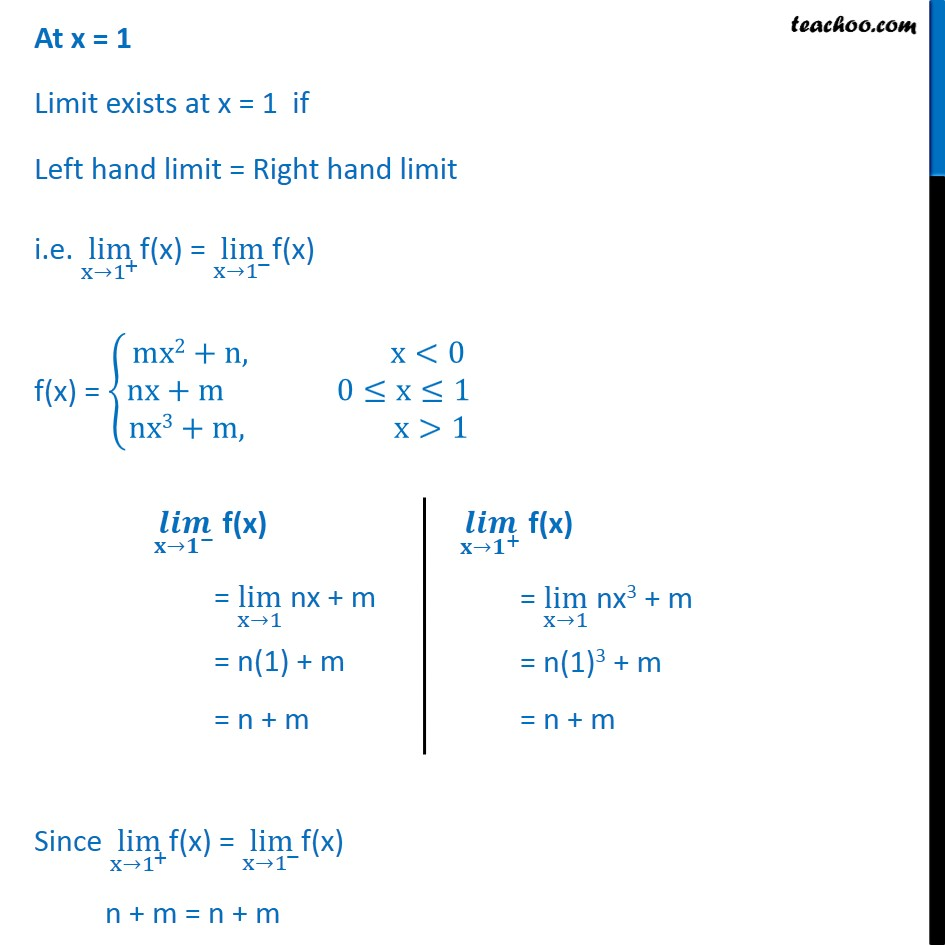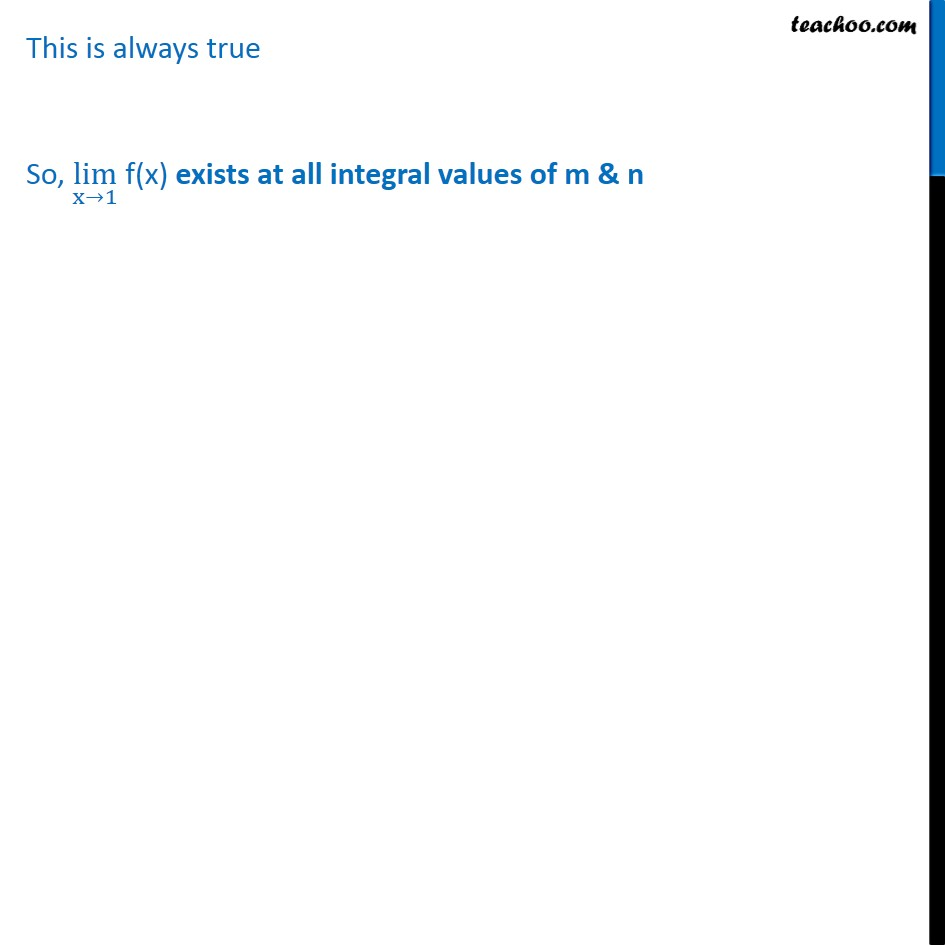1. Class 11
2. Important Question for exams Class 11
3. Chapter 13 Class 11 Limits and Derivatives

Transcript

Ex 13.1, 32 If f(x) = mx2+n, x<0 nx+m 0 x 1 nx3+m, x>1 . For what integers m and n does lim x 0 f(x) and lim x 1 f(x) exist? Given limit exists at x = 0 and x = 1 At x = 0 Limit exists at x = 0 if Left hand limit = Right hand limit i.e. lim x 0 + f(x) = lim x 0 f(x) f(x) = mx2+n, x<0 nx+m 0 x 1 nx3+m, x>1 Finding lim x 0 f(x) & lim x 0 + f(x) Since lim x 0 + f(x) = lim x 0 f(x) m = n So, for lim x 0 f(x) to exist, we need m = n , where m, n are integers At x = 1 Limit exists at x = 1 if Left hand limit = Right hand limit i.e. lim x 1 + f(x) = lim x 1 f(x) f(x) = mx2+n, x<0 nx+m 0 x 1 nx3+m, x>1 Since lim x 1 + f(x) = lim x 1 f(x) n + m = n + m This is always true So, lim x 1 f(x) exists at all integral values of m & n

Chapter 13 Class 11 Limits and Derivatives

Class 11
Important Question for exams Class 11